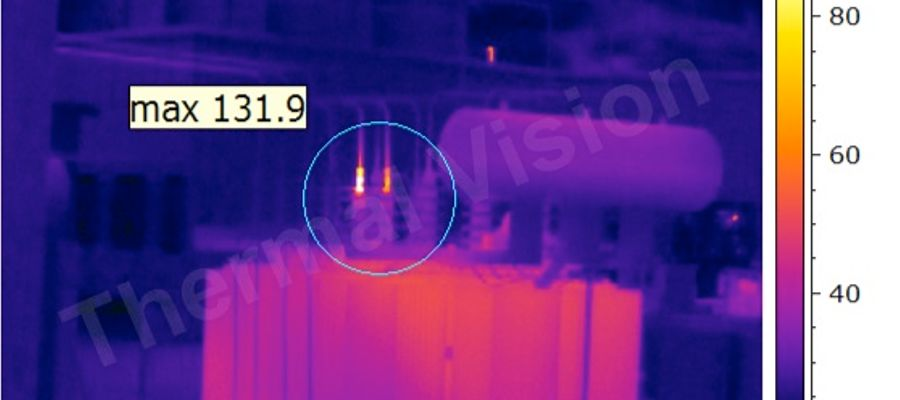# Thermography 1

## IR quiz### Quiz Questions

• 1.
• A.
Isaac Newton
• B.
Galileo
• C.
William Herschel
• D.
Max Planck
• 2.
The law describing the relationship between a bodies ability to emit, and its ability to absorb is called
• A.
Stephan-Boltzmann law
• B.
Kirchoff’s law
• C.
1st law of thermodynamics
• D.
2nd law of thermodynamics
• 3.
If the emissivity on an opaque object equals 0.7, what is its reflectivity?
• A.
0.7
• B.
1
• C.
0.3
• D.
0.4
• 4.
In the electromagnetic spectrum where is the infrared region located?
• A.
Between visual and microwave regions
• B.
• C.
Between visual and UV regions
• D.
Between UV and x-ray regions
• 5.
Which statement about emissivity and colour is correct?
• A.
Emissivity is dependent on colour
• B.
White has a higher emissivity than any other colour
• C.
Black has a higher emissivity than any other colour
• D.
Colour is independent of emissivity
• 6.
For an object with ε=0.1, and τ=0.1, what is ρ?
• A.
0.1
• B.
0.2
• C.
0.3
• D.
0.8
• 7.
Which statement below is correct about heat
• A.
Heat does not exist below 0°C
• B.
Heat flows from a low to a high temperature region
• C.
Heat only flows if there is thermal equilibrium
• D.
Heat requires a temperature difference to flow
• 8.
Which standard below relates to certification of thermographers?
• A.
ISO 18434
• B.
BS/EN 13187
• C.
BRE 176
• D.
ISO 18436
• 9.
The 2nd law of thermodynamics states
• A.
Heat flows from cold to hot
• B.
Heat flows from hot to cold
• C.
Heat does not flow between objects in equilibrium
• D.
Heat flows between objects in equilibrium
• 10.
What of the following cannot be changed later in the software?
• A.
Focus, dynamic range & image composition
• B.
Distance, air temperature & relative humidity
• C.
Level, span & emissivity
• D.
None of the above can be changed
• E.
All of the above can be changed
• 11.
Of the 4 tested materials listed below, which one is most suitable as an infrared mirror?
• A.
ε=0.92. τ=0.
• B.
ε=0.02. τ=0.8
• C.
ε=0.4. τ=0
• D.
ε=0.8. τ=0
• 12.
What camera settings correct for atmospheric effects?
• A.
Reflected temperature, distance & range
• B.
Level, span & dynamic range
• C.
Emissivity, reflectivity & transmissivity
• D.
Distance, air temperature & relative humidity
• 13.
What are infrared camera lenses generally made from?
• A.
Glass
• B.
Germanium
• C.
Gallium arsenide
• D.
Amorphous silicon
• 14.
Which of the following standards provides guidance for the contents of a thermography report?
• A.
ISO 18436 only
• B.
ISO 18434 only
• C.
Both ISO 18436 & ISO 18434
• D.
Neither
• 15.
What is the main difference between quantitative and qualitative thermography?
• A.
Qualitative uses temperature measurement
• B.
Quantitative uses temperature measurement
• C.
Both qualitative and quantitative use temperature measurement
• D.
Neither qualitative nor quantitative use temperature measurement

• 1.
Result 1